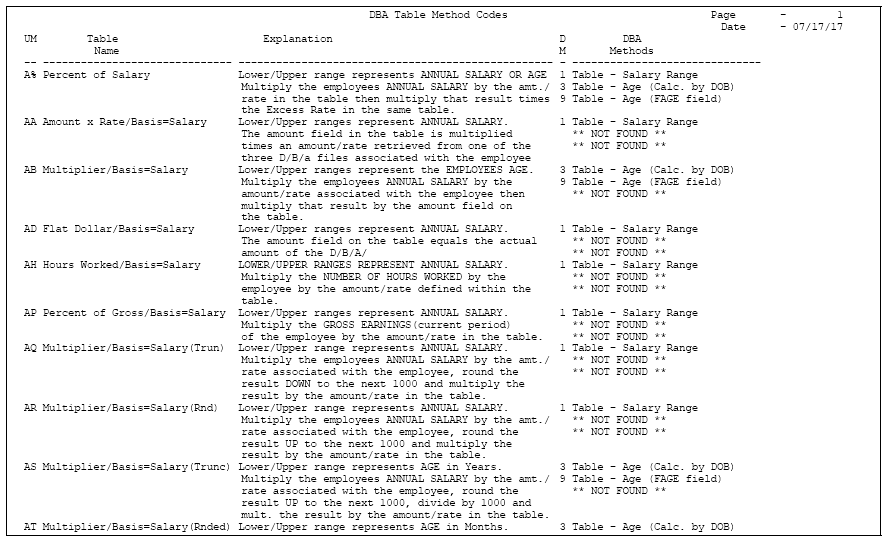49 Set Up Calculation Table Information

This chapter contains these topics:

49.1 Setting Up Calculation Table Information

You set up calculation tables to define the parameters the system uses to calculate DBAs. After you set up your calculation tables, you can review the Calculation Tables report to verify that the information that you entered on the calculation tables is accurate. After you set up your calculation tables, you attach the table to the DBA by specifying the table code and appropriate table method when you set up a DBA.

Setting up DBA calculation information includes the following tasks:

• Setting Up Calculation Tables

• Attaching Calculation Tables to DBAs

• Reviewing the Calculation Tables Report

• Reviewing the DBA Table Method Codes Report

49.2 Setting Up Calculation Tables

From Payroll Master (G07), enter 29

From Payroll Setup (G074), choose Pay/Deductions/Benefits

From Pay/Deductions/Benefits (G0742), choose Calculation Tables

You set up calculation tables to define the parameters for calculating DBAs that are based on variable values. You define valid ranges and amounts that are a function of certain method codes.

Calculation tables contain user defined information to more completely define the standard DBA methods of calculation. Calculation tables provide the following features:

• User defined tables

• Calculations based on values that vary from employee to employee

When you define a calculation table, you must first determine what the calculation is based on, for example:

• Age

• Annual salary

• Months of service

• Hours worked in a pay period

After you have defined the calculation table, you must determine the appropriate method of calculation. The calculation tables only work with certain method of calculation codes. The method of calculation indicates what the ranges in the table represent, such as age ranges or salary ranges. The method of calculation codes include the following:

Code Description
0 Withholding periods
1 Salary range
2 Date range
3 Age range (calculated by date of birth or DOB)
4 Hours worked
5 Pieces produced
6 Variable periods
8 Gross pay range
9 Age (calculated as of the date you enter in the Employee Age field on Pay Rate Information)
Z Spouse's age by actual DOB

For example, to base a DBA on employee age, you can use codes 3 or 9 for the method of calculation.

Browse through the list of table methods and choose one appropriate to the method of calculation. Read the description to find one that matches how you want the system to perform the calculation.

You can enter multiple ITD limits for a single accrual. Accruals with ITD limits also take into account all current pay period activity related to the accrual. If current pay period taken amounts bring an employee below the ITD limit, the accrual calculates in that same payroll cycle. The accrual will stop calculating if the employee is at or above the ITD limit. If vacation time is taken during the payroll to reduce the employee's current vacation amount below the ITD limit, the accrual calculates again up to the ITD limit.

Note:

The ITD limits you enter in the calculation table override the ITD limit in the F19 (Previous) screen of the accrual.

To set up calculation tables

On Calculation Tables

Figure 49-1 Calculation Tables screen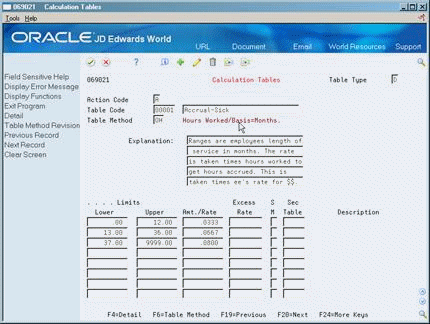Description of ''Figure 49-1 Calculation Tables screen''

1. Complete the following fields:

• Table Type

• Table Code

• Table Method

• Explanation

2. To define the parameters of the table, complete the following fields:

• Lower Limit

• Upper Limit

• Amount or Rate

3. Complete the following optional fields:

• Excess Rate

• Secondary Calculation Method (SM)

• Secondary Calculation Table

4. Repeat steps 2 and 3 as often as necessary to define all the parameters needed for the table.

To set multiple Inception-To-Date limits

You can associate multiple inception-to-date limits with a single accrual. These limits override the ITD limit in the F19 window of the accrual.

On Calculation Tables

Figure 49-2 Calculation Tables screen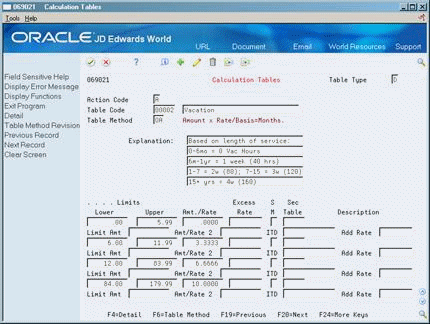Description of ''Figure 49-2 Calculation Tables screen''

1. Select Detail from the Navigation bar to open the fold.

2. Enter ITD limit(s) for each range in the calculation table.

3. Press Enter.

Field Explanation
Table Type A code that defines the purpose of the table. Valid values are:

D – The system uses the table to calculate DBAs.

R – The system uses the table to determine limits for rolling over sick and vacation accruals.

Table Code A numeric code that identifies this table in the Table file (F069026).
Table Method A code that specifies the method the system uses to calculate the DBA.

Form-specific information

This code indicates the type of information in the Amount field, for example, hours or dollars.

Lower The lower or minimum amount to be compared.
Upper Limits The upper or maximum amount to be compared.
Amt./Rate The amount or rate the system uses to calculate a DBA. When you enter 1, 2, 3, 4, 5, or 6 as the method of calculation, you must enter a value in this field to use in the calculation in conjunction with the basis table.
Rate A rate that the system applies to the amounts that exceed the table defined amount.
S M A user defined code (07/DS) that indicates which method the system uses to calculate DBAs.

Form-specific information

The secondary method the system can use in calculating the DBA. When the system uses the secondary method, the first table serves as an eligibility table. Eligibility on the primary table is based on salary range, date range, and age range, in that order.

Sec Table A code which specifies the method under which the DBA is to be calculated.

This calculation table serves as the secondary calculation table for the system. You must enter a code in this field if you enter a code in the secondary method field.

49.2.1 What You Should Know About

Topic Description
Viewing table methods online Choose the Table Method function to locate information on individual table methods.

The table method explains which fields in the table the system uses for calculations.

Zero amounts You do not need to include a line in the table for zero amounts. For example, if vacation is not earned in the first year of employment, you do not need to include that year on the first line of the calculation table. The first line could start with 2.00 as the lower limit.
Table method DBAs DBAs entered at the employee level override DBA amounts or rates defined at the group or setup level unless it is a table method DBA. A table method DBA is a calculation table that has a table method code attached to it specifying how the system calculates the DBA. If you reference a DBA that has a table method code attached, you cannot override the amount. The system does not recognize another method to calculate a DBA when a table method code is attached.
Override of DBA Limit The information on the calculation table overrides any information entered on DBA Limit.

49.3 Attaching Calculation Tables to DBAs

From Payroll Master (G07), enter 29

From Payroll Setup (G074), choose Pay/Deductions/Benefits

From Pay/Deductions/Benefits (G0742), choose DBA Setup

After you set up your calculation tables, attach the table to the DBA by entering the table code on DBA Setup.

To attach calculation tables to DBAs

On DBA Setup

Figure 49-3 DBA Setup screen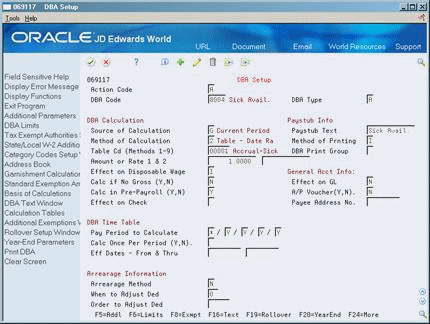Description of ''Figure 49-3 DBA Setup screen''

1. Complete the following fields:

• Method of Calculation

• Table Code

2. Complete the remaining steps for setting up a DBA.

49.3.1 What You Should Know About

Topic Description
Attaching calculation tables to multiple DBAs In most cases you attach a calculation table to a single DBA. JD Edwards World recommends that you make the table code the same as the DBA code. You can attach the same calculation table to more than one DBA.

49.4 Reviewing the Calculation Tables Report

From Payroll Master (G07), enter 29

From Payroll Setup (G074), choose Pay/Deductions/Benefits

From Pay/Deductions/Benefits (G0742), choose Calculation Tables

The Calculation Tables Report provides a listing of the calculation tables by table code. Review this report to verify that the information you entered on each calculation table is accurate.

Figure 49-4 Calculation Tables Report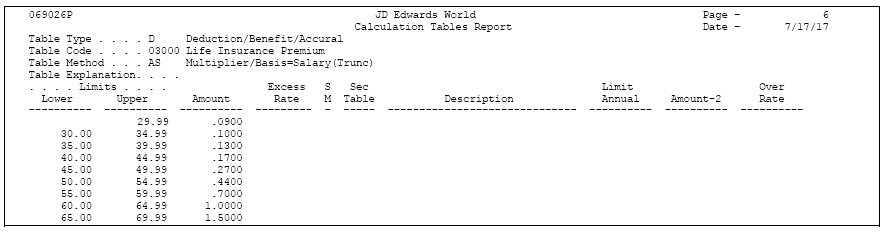Description of ''Figure 49-4 Calculation Tables Report''

49.5 Reviewing the DBA Table Method Codes Report